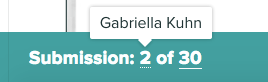How to manage “best out of” Gradescope exams and other assessments, where students can answer all of the questions, but are only the best x marks are counted.

Example:

Section A (compulsory) and Section B (best x answers out of y questions – e.g. best 2 out of 4).

How can you adjust the total mark for the paper, while keeping the marks for the individual questions?

## Workflow

1. List all of the questions on the Edit Outline page with the correct point values.

Before grading submissions, add a new, additional question to the Outline. Title it something like “Drop question”.
Make the max point value of that question -n (i.e. a negative value), where n is the point value of the question you’re dropping.

Note that all questions in the group must have the same max point value individually for this to work. For example, if students are asked to answer 6 questions but only the five highest scores will count, for a maximum score of 25 points on that section, you’d list all six questions on the outline page with “5” as the point value, and make the point value of the drop question “-5”.  If students are asked to answer four out of the six 5-point questions, you’d set the drop question value to -10.

2. Grade all student submissions for all questions as normal.
4. Go to Drop Question from the Grade Submissions page.
In Rubric Settings, disable the point floor and ceiling and set the question type to positive scoring.
Apply a rubric item whose point value is “-n” where n is the student’s lowest score (or the sum of the student’s two lowest scores if you’re dropping two questions).
As you’re doing this, you can see the name of the student you’re grading by hovering over the submission number in the lower left corner of the grading page:As an example for step 4, let’s say the student’s scores for the six questions (in the example described in step 1) are 5, 5, 4, 3, 5, and 5.
The lowest score is 3.
So for the Drop Question, the instructor would apply a -3 point value rubric item to the student (or a -3 point submission-specific adjustment), and the student’s score for the drop question would be -3/-5.

If you want to drop the two lowest scores, you’d apply a -7 point adjustment and the student’s score for the drop question would be -7/-10.

### Alternative Step 4) for Exams

Note that, for exams, there is no requirement to show students their marks in either Gradescope or Blackboard, so it is perfectly acceptable to manage this type of assessment in Excel, and then enter the marks into RISIS.

Use Excel to

• find the lowest score
• calculate correct total score.

Leave the raw marks per question as they are in Gradescope.

Then

• upload the total to the Blackboard Grade Centre (involves some work to make sure the marks from the Gradescope spreadsheet are entered against the correct student on the Blackboard spreadsheet).
• or enter the marks directly into RISIS (requires use of VLOOKUP).